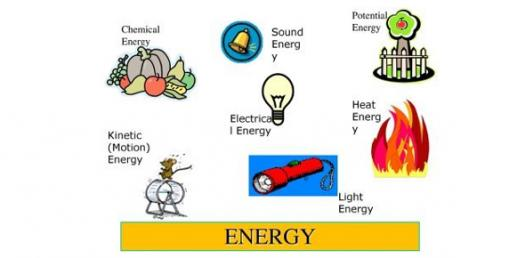# Science Energy Quiz For 9 Year's Students

10 Questions | Attempts: 3589
ShareSettingsYear 9 Science students

• 1.
Which of the following are NOT types of energy
• A.

Light

• B.

Sound

• C.

Electricity

• D.

Wood

• 2.
when you eat an ice cream, your body uses the __________ energy in it
• 3.
when a fan heater is going, what kind of energy transformation (change from one type to the other) is happening?
• A.

Electrical energy is being transformed into light energy

• B.

Electrical energy is being transformed into heat energy

• C.

Heat energy is being transformed into light energy

• D.

Heat energy is being transformed into electrical energy

• 4.
Electricity involves the movement of one of the particles in an atom. (subatomic particles)  The subatomic particle involved in electricity is the ...(HINT look at the word 'electricity')
• A.

Neutron

• B.

Proton

• C.

Electron

• 5.
The law of conservation of energy states that:In a closed system, the amount of energy remains constant over timeIn other words, energy can change from one kind to another, but none of it disappears or appears from nowhere.In the sentence above, the word 'conservation' means....
• A.

Closed

• B.

Keeping safe

• C.

Keeping the total amount the same

• 6.
When we use a battery to power a crazed pink mouse toy (which jumps up and down), we are turning chemical energy into electrical energy, and then we are turning (transforming) the electrical energy into _____________ energy.
• A.

Kinetic (movement)

• B.

Light

• C.

Heat

• 7.
Sticking with the crazed mouse toy, I am now going to add the fact that it has springs in its feet. What type of energy is involved in the springs?
• A.

Elestic potent energy

• B.

Alastic potent energy

• C.

Ellatic potential energy

• D.

Elastic potential energy

• 8.
The unit of energy is the ...(Unit is what we measure something in, e.g. the unit for time is the second)
• A.

Jewel

• B.

Jole

• C.

Joule

• D.

Jail

• 9.
How many joules are in a kilojoule?
• A.

100

• B.

1000

• C.

10

• D.

10, 000

• 10.
The average teenage boy needs 10,000 to 15,000kJ a day. (Girls need less)How many kJ (kilojoules) do you think are in a small glass of lemonade?
• A.

• B.

• C.